Janson inequality

There are a couple of inequalities referred to as "Janson inequality" . They provide exponential upper bounds for the probability that a sum of dependent zero-one random variables (cf. also Random variable) equals zero. The underlying probability space corresponds to selecting a random subsetof a finite set, where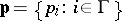, in such a way that the elements are chosen independently with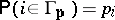for each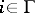.

Let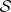be a family of subsets ofand, for every, let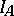be equal to one ifand be zero otherwise. Then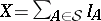counts those elements ofwhich are entirely contained in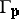. Set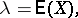Then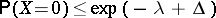(a1)

and, which is better for,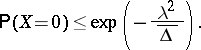(a2)

Research leading to these inequalities was motivated by a ground-breaking proof of B. Bollobás [a2], who, in order to estimate the chromatic number of a random graph, used martingales (cf. also Graph colouring; Graph, random; Martingale) to show that the probability of not containing a large clique is very small. Bollobás presented his proof at the opening day of the "Third Conference on Random Graphs (Poznań, July 1987)" . By the end of the meeting S. Janson found a proof of inequality (a1) based on Laplace transforms (cf. also Laplace transform), while T. Łuczak proved a related, less explicit estimate using martingales. The latter result was restricted to a special, though pivotal, context of small subgraphs of random graphs. Soon after, R. Boppana and J. Spencer [a1] gave another proof, resembling the proof of the Lovász local lemma, of the following version of (a1):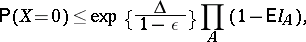(a3)

where. This version puts emphasis on comparison with the independent case.

Under modest symmetry conditions, inequality (a2) appeared in [a3], while the general version was proved in [a4]. See also [a6] for another proof of (a2), but with an extra factorin the exponent.

The quantityis a measure of the pairwise dependence between thes. If, then the exponents in (a1) and (a2) are both equal to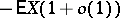, matching asymptotically a lower bound obtained via the FKG inequality, provided further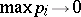.

Example.

Letbe an integer,, and letbe the set of all two-element subsets of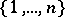. With all,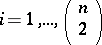, the random subsetis a random graph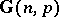. Letbe the family of all triples of pairs of the form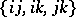,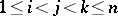. Thenis the number of triangles in,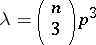and. Thus, if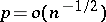, then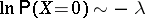, while for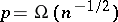, inequality (a2) yields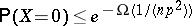. As long as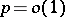,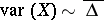, and the above exponential bounds strengthen the polynomial bound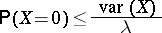obtained by the method of second moments, i.e. by a corollary of the Chebyshev inequality. To illustrate the strength of (a1), fix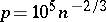and assume thatis divisible by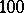. Then (a1) easily implies that with probability tending to one, more thanof the vertices ofare covered by vertex-disjoint triangles. Indeed, otherwise there would be a subset of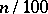vertices spanning no triangle. By (a1), the probability that this may happen is smaller thanIn 1990, Janson [a4] generalized inequality (a2), obtaining an estimate of the lower tail of the distribution of. With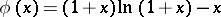, for,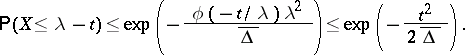(a4)

Whenconsists of mutually disjoint sets,is a sum of independent zero-one random variables and (a4) coincides with the (one-sided) Chernoff bound. No corresponding upper tail estimate is true in general.

Also in 1990, W.C.S. Suen [a7] proved a related inequality, which remains valid for a general probability space (and not only for random subsets). In his setting,is a sum of arbitrary indicators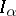, and the bound hinges on the dependency graph induced by them. Suen's inequality has been revived and extended in [a5].

Fore more details on this subject see [a8].